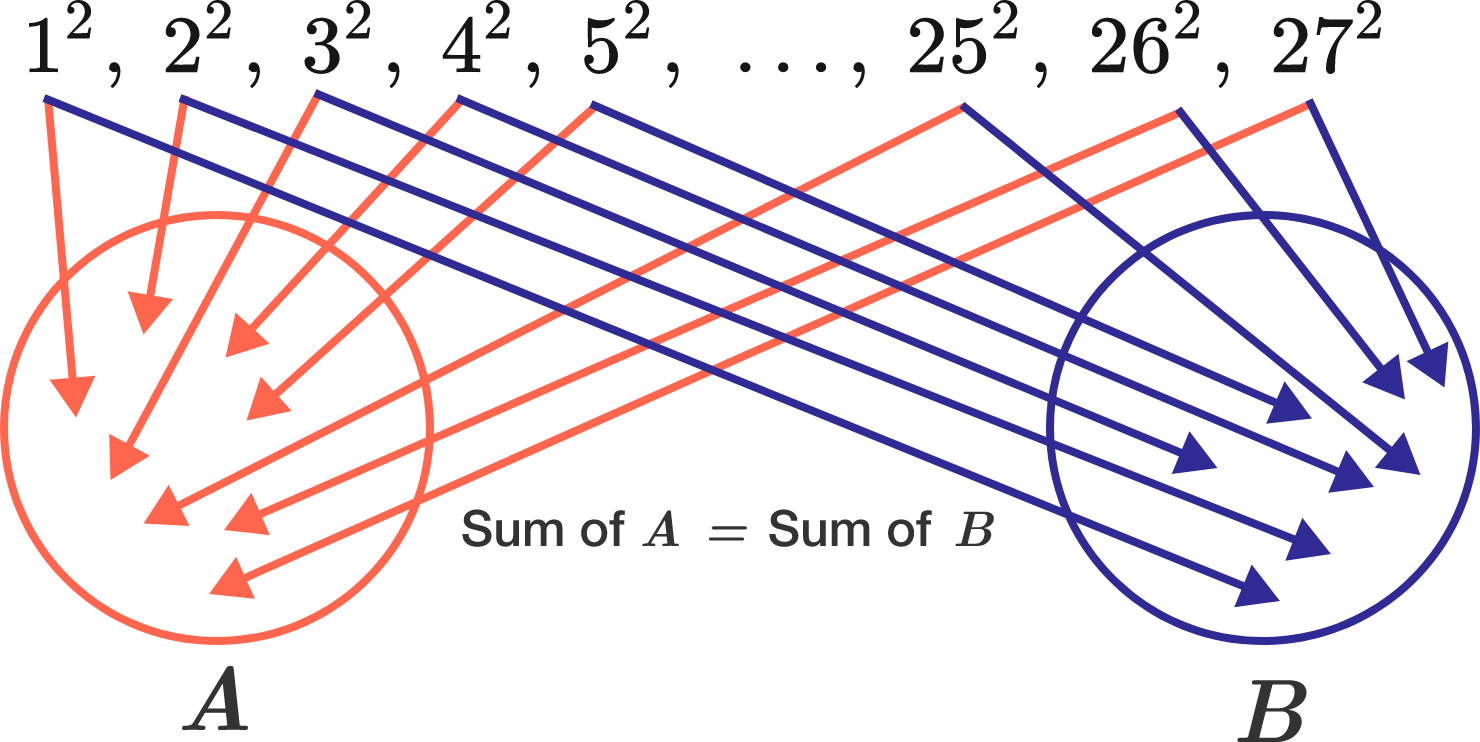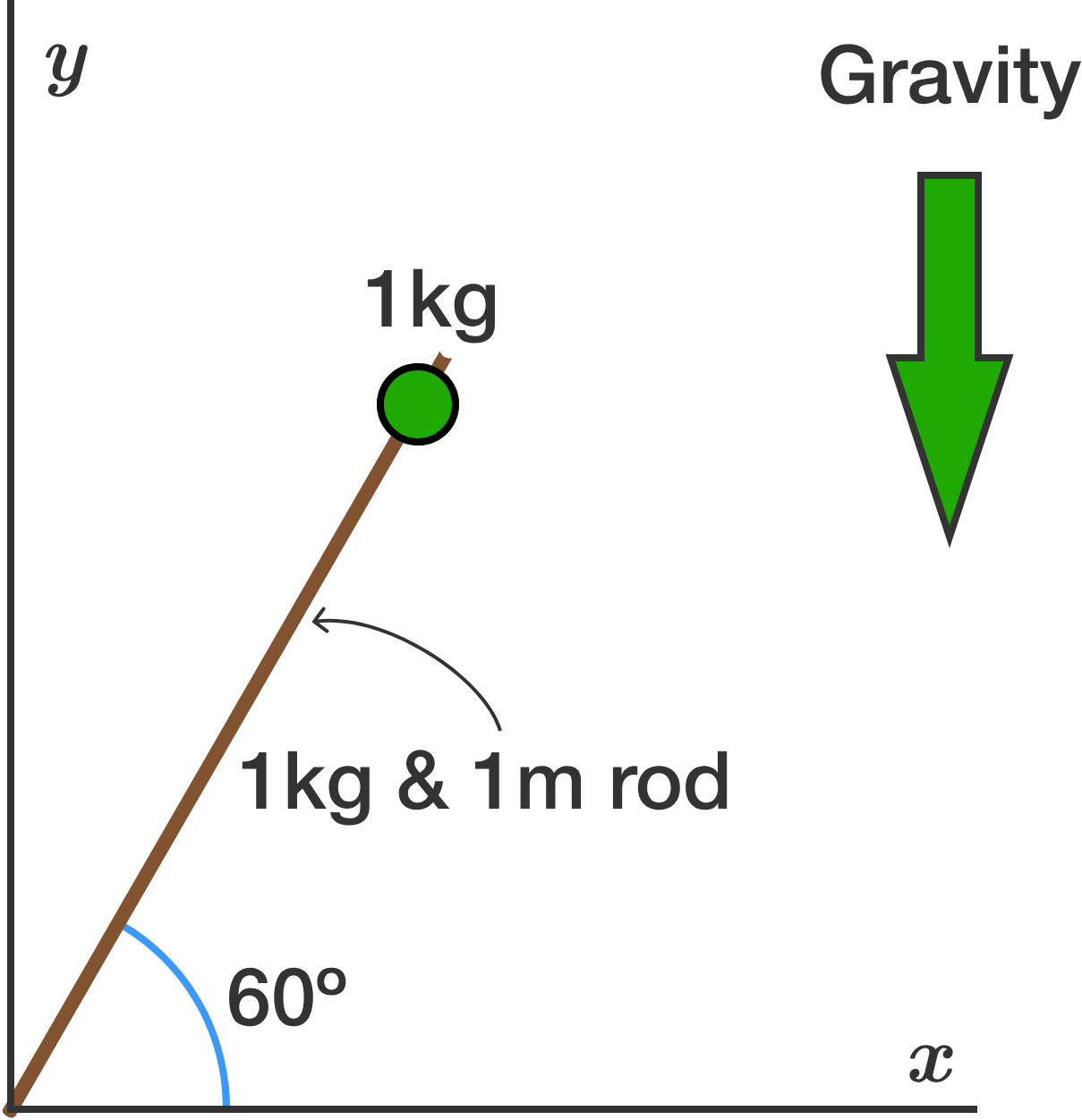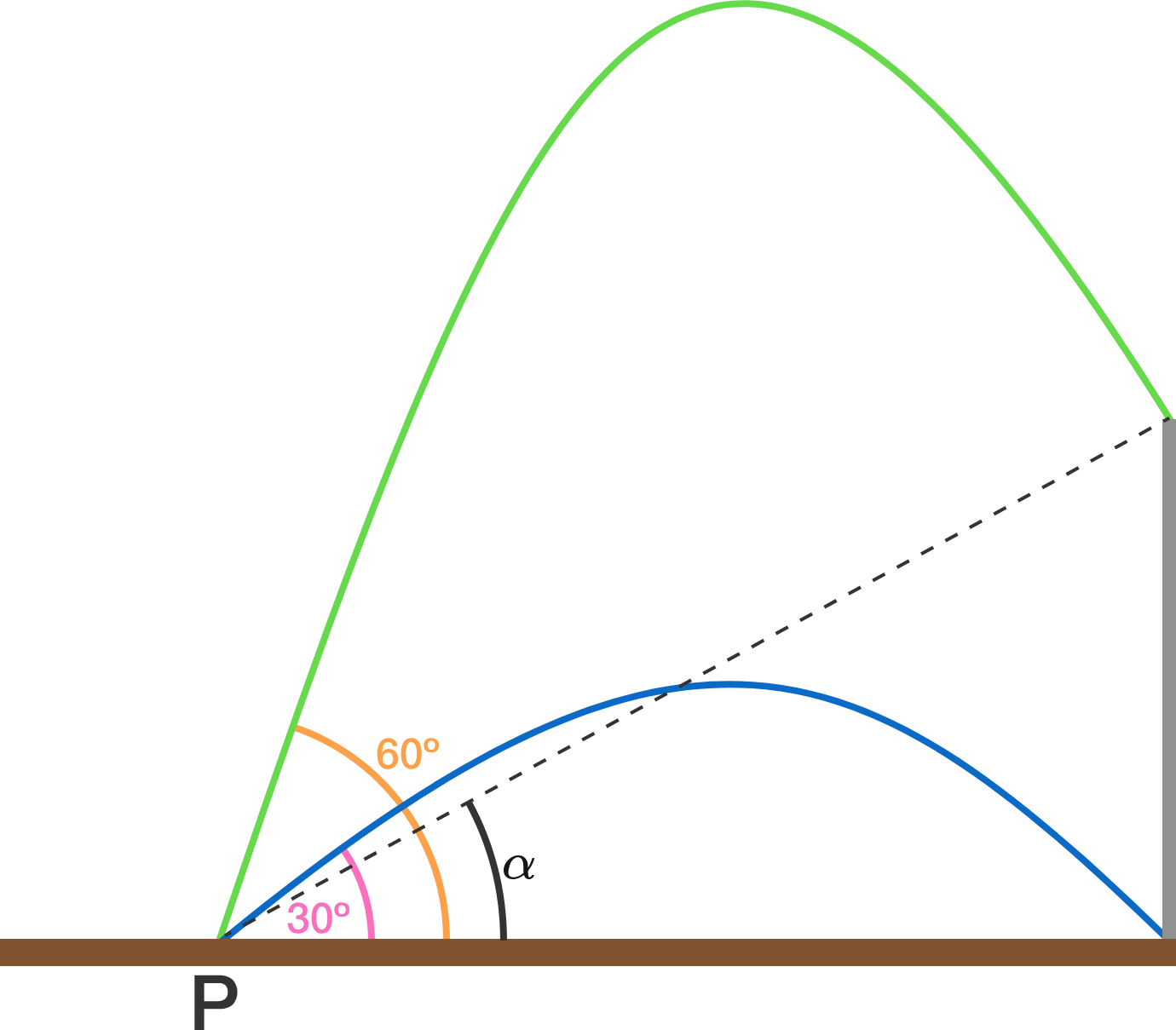# Problems of the Week

Contribute a problem

Is it possible to place every one of the following numbers $1^2,\ 2^2,\ 3^2,\ 4^2,\ 5^2,\ \dots,\ 25^2,\ 26^2,\ 27^2$ into either of the two regions $A$ and $B$ below such that the sums of the numbers in each region are equal?A mathlete has been tracking the percentage of problems he has gotten right so far this season.

Let $n$ be the number of problems given to him, and $m$ the number he has gotten correct.

Find the sum of all $0 such that if at some point in the season $\frac{m}{n}, and at some later point in the season $\frac{m}{n}>q$, then there will always be a third point in the season where $\frac{m}{n}=q.$

If you believe that no such $q$ exists, submit $-1$ as your answer.

Clarification: Although it is true that any rational $q$ may be obtained after the first two points, the problem requires that this rational $q$ must exist at some point in the season. In particular, it might be helpful to analyze points in the season between the two points satisfying the first two conditions.

Let $x_n$ be a positive root of the equation $x^n = x^2 + x + 1.$

Find $\displaystyle \exp\Big(\lim_{n \to \infty} n\left(x_n - 1\right)\Big).$


Notation: $\exp( a ) = e^a$A $\SI{1}{\meter}$ rod of uniform composition and total mass $\SI{1}{\kilo\gram}$ has one end hinged at the origin. A $\SI{1}{\kilo\gram}$ bead is placed at the other end and is free to slide along the rod (if it reaches the open end, it will separate from the rod).

The bead and rod are prepared so that the bead sits near the open end of the rod which makes an angle of $\theta=60^\circ$ with the horizontal.

If this system is released from rest at time zero, at what time (in seconds) does the bead slide off of the rod?

Assumptions:

• $g = \SI[per-mode=symbol]{10}{\meter\per\second\squared}.$

There is a vertical pole perpendicular to the horizontal plane. From point $P$ on the plane, 2 projectiles are fired simultaneously at different velocities. The first projectile is fired at an angle of $30^{\circ}$ and it hits the foot of the pole. The second projectile is fired at an angle of $60^{\circ}$ and it hits the top of the pole.It is further known that the projectiles hit the pole at the same time.

If the angle subtended by the pole from $P$ is $\alpha$, find $\tan \alpha$ to 3 decimal places.


Details and Assumptions:

• Air resistance is negligible.
• A gravitational pull is present.
×